RSWebinar: Designing Cost-Effective Ground Improvement Solutions with Settle3 | June 7th, 2023 Register Now

# Define Hydraulic Properties

If you are performing a groundwater analysis, the Define Hydraulic Properties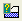option is used to specify the permeability characteristics for your materials. Material permeability is required by the finite element analysis, in order to solve the groundwater problem (i.e. determine the flow and pressure distribution throughout the model).

• Hydraulic Properties can only be defined for finite element groundwater analysis (i.e. Groundwater Method = Steady State FEA or Advanced Groundwater = Transient in Project Settings).
• Also, the Analysis Mode must be set to Groundwater (steady-state or transient) so that the groundwater modelling options are available in the Slide2 menu and toolbar.
• In this case, the Define Hydraulic Propertiesoption will be available in the toolbar and the Properties menu.
• The materials in the Define Hydraulic Properties dialog, correspond to the materials in the Define Material Properties dialog (i.e. the dialog in which you define the strength properties of your materials, when the Analysis Mode = Slope Stability).
• The material names and colours which appear in the Define Hydraulic Properties dialog, are those which are specified in the Define Material Properties dialog (the names and colours CANNOT be edited in the Define Hydraulic Properties dialog).

## Saturated Permeability

A saturated permeability, Ks, must always be specified for a material. However, note that:

• If you are using a User Defined Permeability Function (see below for details), then the saturated permeability is specified as the first point of the permeability function (i.e. permeability at matric suction = 0). In this case, the saturated permeability option, Ks, will be disabled.
• For all other permeability function models (i.e. Simple, van Genuchten etc), the saturated permeability Ks is enabled.

## Anisotropic Permeability Parameters

Anisotropic permeability may be specified for a material (i.e. permeability varies with direction), by specifying a Factor and Angle, which defines the relative permeability in two orthogonal directions.

• K2 / K1 is the factor which specifies the relative permeability in the direction orthogonal to the K1 direction. NOTE that the K1 permeability is the "primary" permeability defined by the Saturated Permeability Ks, and the unsaturated permeability model you have defined in the dialog.
• K1 Angle is the angle which specifies the direction of the K1 permeability. The K1 Angle is specified relative to the positive X (horizontal) direction, as indicated by the picture in the dialog.

EXAMPLE: if K2 / K1 = 0.5 and K1 Angle = 0, then the permeability in the HORIZONTAL direction = Ks (in the saturated zone), and the permeability in the VERTICAL direction = 0.5 * Ks (in the saturated zone).

## Transient Analysis Parameters

The following parameters must be defined when computing a transient analysis. They are used to define the Soil Water Characteristic Curves (SWCC) when computing unsaturated soil permeabilities.

• WC sat: the saturated water content which is equivalent to porosity.
• WC res: the water content for which the gradient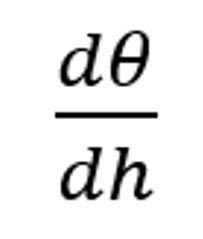becomes zero (where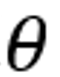is the volumetric water content).
• mv: Coefficient of Volume Compressibility. If the box is left unchecked the default grayed out value is used in the analysis. Note that this value should always be entered, and the checkbox is only present for backwards compatibility.

## Permeability Models

The following models are available in Slide2 for describing the permeability in the unsaturated zone as a function of matric suction.

• Brooks and Corey
• Fredlund and Xing
• Gardner
• Simple
• Van Genuchten
• User-defined

A user-defined function can be defined by selecting the New button. See below for details about each model.

Plot button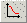After entering the parameters for the selected model, you can select the Plot button to view the actual permeability function which is defined by the model and the parameters you have entered.

Pick button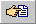If you select the Pick button in the dialog, you will see another dialog, from which you can select from a list of materials, with representative parameters for the selected model. (References for the data are provided in the dialog). If you select OK in this dialog, the currently selected parameters will be automatically loaded into the Define Hydraulic Properties dialog.

### Simple

With the Simple model, an unsaturated permeability function is automatically determined by Slide2 based on the magnitude of the saturated permeability Ks and the selected Soil Type. To view the function determined by Slide2, you can select the Plot button in the dialog. For the Simple model, you can choose one of the following Soil Types:

• General
• Sand
• Silt
• Clay
• Loam

For the General soil type, the unsaturated permeability simply decreases by an order of magnitude within the initial range of matric suction values, and then remains constant for higher values of suction.

For specific soil types (Sand, Silt, Clay or Loam) average curves have been determined from typical literature values.

### Brooks and Corey

The following equations define the Brooks-Corey permeability function (Brooks and Corey, 1964):

 For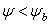: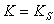Eqn. 1a For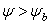: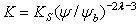Eqn. 1b where: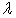= Pore size index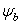= bubbling pressure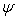= matric suction K = permeability Ks = saturated permeability

### Fredlund and Xing

The following equation defines the Fredlund-Xing permeability function (Fredlund and Xing, 1994):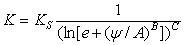Eqn.2 where: A, B and C are the model parameters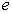= 2.7182818 …= matric suction K = permeability Ks = saturated permeability

### Gardner

The following equation defines the Gardner permeability function (Gardner, 1956):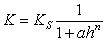Eqn.3 where: a and n are the model parameters h = pressure head (suction) K = permeability Ks = saturated permeability

### Van Genuchten

The following equations define the van Genuchten permeability function (van Genuchten, 1980):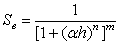Eqn.4a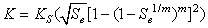Eqn.4b where: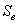= degree of saturation h = pressure head (suction)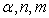are the model parameters K = permeability Ks = saturated permeability NOTE: the parameters m and n are related as follows: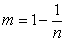Eqn. 4c Eqn.4c

However, you can specify a value of m which is independent from n, by selecting the Custom m checkbox, and entering a value for m.

### User Defined

For complete flexibility in defining permeability functions, a User Defined Permeability Function can be defined as follows:

1. Select the New button in the Define Hydraulic Properties dialog.
2. You will see the User Defined Permeability Function dialog.
3. Enter a Name for the function.
4. Enter the Matric Suction and Permeability values which define the required function. As you enter the data, the graph at the right of the dialog will display the function you are defining.
The data points on the graph can be moved graphically by clicking on them with the mouse and dragging.
1. When all data has been entered, select OK, and you will be returned to the Define Hydraulic Properties dialog.
2. In the Define Hydraulic Properties dialog, the Name of the User-Defined Permeability Function you have defined will now appear in the Model drop-list. It can be selected at any time, just like any of the other permeability models.

Edit

To modify an existing User Defined Permeability Function, select the Name of the function from the Model list, and select the Edit button. You can then edit the data which defines the function, in the User Defined Permeability Function dialog.

Delete

To delete an existing User Defined Permeability Function, select the Name of the function from the Model list, and select the Delete button.

Copy

To create a copy of an existing User Defined Permeability Function, select the Name of the function from the Model list, and select the Copy button. Enter a new name in the dialog which appears, and select OK. A new function will automatically be created, and the Name will appear in the Model list.

Import/Export

User-Defined Permeability Functions in Slide2 can be saved in their own separate file, if desired.

• To save/export a User Defined Permeability Function file, select the Export button in the User Defined Permeability Function dialog. These files have a *.FN5 filename extension.
• To import a User Defined Permeability Function file, select the Import button in the User Defined Permeability Function dialog.